# Analytical reasoning test-2

11)

In each of the following questions, count the number of squares in the figure.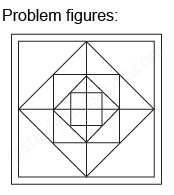12)

In each of the following questions, count the number of squares in the figure.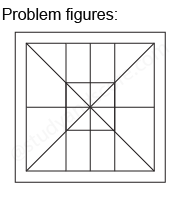13)

In each of the following questions, count the number of squares in the figure.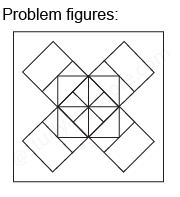14)

In each of the the following question, count the number of triangles and squares in the given figure.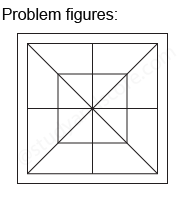15)

What is the minimum number of colours required to fill the spaces in the diagram without any two adjacent spaces having the same colour?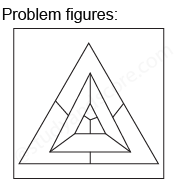--Share with your friends --